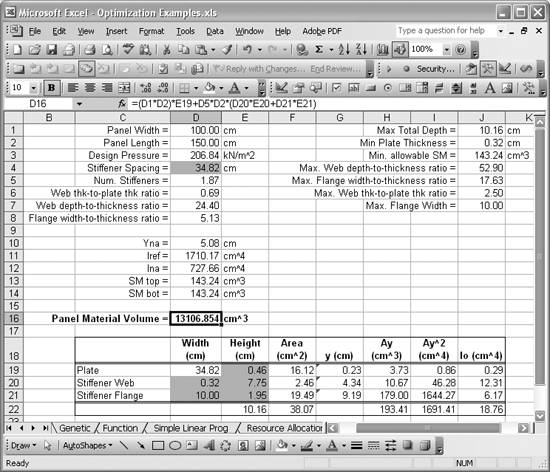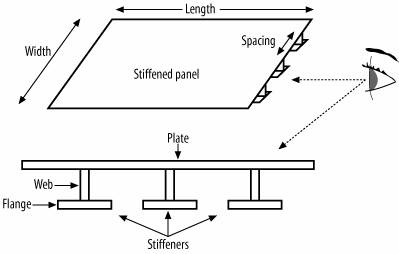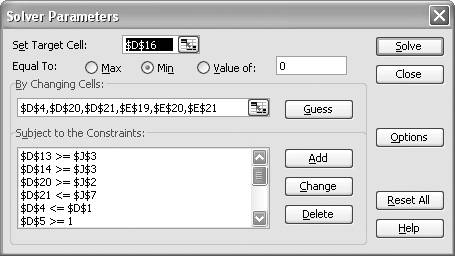# Optimizing Engineering Design Problems

Problem

You're working on an engineering design problem and would like to see how you can leverage Excel to find an optimum design solution.

Solution

Model your design problem in Excel as an optimization problem and then use Solver to find a solution.

Discussion

It is rather straightforward to model many different engineering design problems in Excel. Many engineers do so on a daily basis. Moreover, once you have a model set up in Excel, it's a small step to use Solver to optimize that model. Take a look at the spreadsheet in Figure 13-12.

Figure 13-12. Engineering design optimization exampleThis spreadsheet represents a typical structural engineering design task. I don't want to go too far describing all the structural engineering background relevant to this problem; but I'll give you an overview so you can get the gist of what we're trying to optimize. Basically, this is a model of a stiffened panel. A stiffened panel is comprised of a plate with beams attached to it to provide strength and rigidity. In this case, the panel is comprised of an aluminum plate, 150 cm long and 100 cm wide, supported all around its edges. The plate also has attached to it a number of T-type stiffeners. Figure 13-13 illustrates this sort of panel.

Figure 13-13. Stiffened panelYou can imagine such a panel forming a portion of a wall of an aluminum building or perhaps the hull of an aluminum boat. The latter case is actually the inspiration for this example.

Boats, especially high-speed boats, are sensitive to weight in terms of performance. So a common task facing naval engineers involves sizing panels like this one so that it has enough strength but is also as light as possible. Sure, you could be conservative and use a really thick plate with a lot of stiffening beams, but that would be expensive and heavy.

You have to use some sort of standard criteria to determine whether such a panel is strong enough. There are such codes (as in rules and regulations, not to be confused with software code) for many types of structures and in this particular case, you can use the standard codes applicable to aluminum boats. These codes require engineers to calculate certain properties of the panel to compare with acceptable values given in the codes. The spreadsheet shown in Figure 13-12 performs just this sort of calculation and comparison.

Cells D1 through D8 contain basic information on the panel and associated stiffeners. The table toward the bottom, cells C18 through J22, contains more specific information on the geometry of the components making up the stiffened panel. This panel also performs the required engineering calculations needed to determine the properties of the stiffened panel. The results of these calculations are contained in cells D10 through D14.

Finally, cells J1 through J7 contain code-related requirements on the properties of the stiffened panel. These cells also contain some practical engineering limits.

The optimization task is to find an optimum combination of plate thickness, stiffener web size, stiffener flange size, and spacing between stiffeners that will yield a panel meeting the code requirements, while at the same time resulting in the minimum achievable weight. Therefore, in terms of optimization, minimizing weight is the objective. All the other requirements become constraints.

Since the material is homogeneous and uniform in this case, we can minimize weight by minimizing the total volume of metal material used to build the stiffeners and panel. The volume is computed by summing the volumes of the plate and stiffeners. Cell D16 contains the volume calculation, and you can see the formula in the formula bar in Figure 13-12. This is our objective function.

The variables we want to change include the stiffener spacing, plate thickness, stiffener web depth and thickness, and stiffener flange width and thickness.

Figure 13-14 shows the Solver model I set up for this problem.

Figure 13-14. Design optimization Solver modelAs expected, cell D16, the objective function, is the target cell, which is set to be minimized. The By Changing Cells textbox includes all of the shaded cells in Figure 13-12 corresponding to the variables we want to vary, as discussed a moment ago.

All the code and design requirements discussed earlier become constraints. (See Chapter 9 for more on setting up Solver models and adding constraints.) For example, the section modulus (SM) of the stiffened panel (SM top and SM bot in the spreadsheet) must be greater than or equal to the Min. allowable SM specified in cell J3. The corresponding Solver constraints are \$D\$13 >= \$J\$3 and \$D\$14 >= \$J\$3, as shown in Figure 13-14. I added similar constraints for each design requirement listed in cells J1 through J7.

Running Solver resulted in the optimum values shown earlier in Figure 13-12. Initially, I tried 50 cm for stiffener spacing and 1 cm for all other optimization variables. Solver readily found a solution with this initial guess. Just to be sure, I tried several other initial guesses; Solver converged on the same solution, so I'm fairly confident that this solution represents a global optimum in this particular design space.

In summary: if you can model a design problem in Excel, you can optimize it in Excel. The trick is in identifying your objective function and the variables you want to change. For highly nonlinear problems, you also have to be careful that you don't converge to a local minimum. You can use the technique I showed you in Recipe 13.4 to mitigate the risk of not finding a global optimum.Excel Scientific and Engineering Cookbook (Cookbooks (OReilly))
ISBN: 0596008791
EAN: 2147483647
Year: N/A
Pages: 206
Authors: David M Bourg

Similar book on Amazon